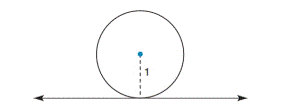Chapter 9.4, Problem 48EElementary Geometry For College St...

7th Edition
Alexander + 2 others
ISBN: 9781337614085

Solutions

Chapter
SectionElementary Geometry For College St...

7th Edition
Alexander + 2 others
ISBN: 9781337614085
Textbook Problem

Sketch the solid that results when the given circle of radius length 1 unit is revolved about the horizontal line that lies 1 unit below the center of that circle.To determine

To find:

The sketch of the solid when a circle revolves around a horizontal line.

Explanation

Approach:

A torus is a surface of revolution generated by revolving a circle in a three dimensional space, about an axis coplanar with the circle. If the axis of revolution does not touch the circle, the surface has a ring appearance and is called a torus of revolution. The torus has a doughnut shape...

Still sussing out bartleby?

Check out a sample textbook solution.

See a sample solution

The Solution to Your Study Problems

Bartleby provides explanations to thousands of textbook problems written by our experts, many with advanced degrees!

Get Started

In problems 17-30, simplify each expression so that only positive exponents remain. 17.

Mathematical Applications for the Management, Life, and Social Sciences

In Exercises 16, simplify the expression. 6. (1+x2)2(2)2x(2)(1+x2)(2x)(1+x2)4

Applied Calculus for the Managerial, Life, and Social Sciences: A Brief Approach

In words, explain what is measured by SS, variance, and standard deviation.

Statistics for The Behavioral Sciences (MindTap Course List)

Evaluate the integral. 31. tan5xdx

Single Variable Calculus: Early Transcendentals